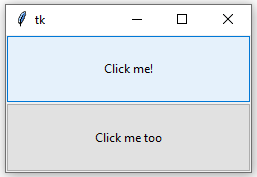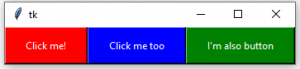Open In App
Related Articles

# Python | pack() method in Tkinter

The Pack geometry manager packs widgets relative to the earlier widget. Tkinter literally packs all the widgets one after the other in a window.  We can use options like fill, expand, and side to control this geometry manager.
Compared to the grid manager, the pack manager is somewhat limited, but it’s much easier to use in a few, but quite common situations:

• Put a widget inside a frame (or any other container widget), and have it fill the entire frame
• Place a number of widgets on top of each other
• Place a number of widgets side by side

Code #1: Putting a widget inside frame and filling entire frame. We can do this with the help of expand and fill options.

## Python3

 `# Importing tkinter module``from` `tkinter ``import` `*` `from` `tkinter.ttk ``import` `*` `# creating Tk window``master ``=` `Tk()` `# creating a Fra, e which can expand according``# to the size of the window``pane ``=` `Frame(master)``pane.pack(fill ``=` `BOTH, expand ``=` `True``)` `# button widgets which can also expand and fill``# in the parent widget entirely``# Button 1``b1 ``=` `Button(pane, text ``=` `"Click me !"``)``b1.pack(fill ``=` `BOTH, expand ``=` `True``)` `# Button 2``b2 ``=` `Button(pane, text ``=` `"Click me too"``)``b2.pack(fill ``=` `BOTH, expand ``=` `True``)` `# Execute Tkinter``master.mainloop()`

Output:Code #2: Placing widgets on top of each other and side by side. We can do this by side option.

## Python3

 `# Importing tkinter module``from` `tkinter ``import` `*``# from tkinter.ttk import *` `# creating Tk window``master ``=` `Tk()` `# creating a Fra, e which can expand according``# to the size of the window``pane ``=` `Frame(master)``pane.pack(fill ``=` `BOTH, expand ``=` `True``)` `# button widgets which can also expand and fill``# in the parent widget entirely``# Button 1``b1 ``=` `Button(pane, text ``=` `"Click me !"``,``            ``background ``=` `"red"``, fg ``=` `"white"``)``b1.pack(side ``=` `TOP, expand ``=` `True``, fill ``=` `BOTH)` `# Button 2``b2 ``=` `Button(pane, text ``=` `"Click me too"``,``            ``background ``=` `"blue"``, fg ``=` `"white"``)``b2.pack(side ``=` `TOP, expand ``=` `True``, fill ``=` `BOTH)` `# Button 3``b3 ``=` `Button(pane, text ``=` `"I'm also button"``,``            ``background ``=` `"green"``, fg ``=` `"white"``)``b3.pack(side ``=` `TOP, expand ``=` `True``, fill ``=` `BOTH)` `# Execute Tkinter``master.mainloop()`

Output:Code #3:

## Python3

 `# Importing tkinter module``from` `tkinter ``import` `*``# from tkinter.ttk import *` `# creating Tk window``master ``=` `Tk()` `# creating a Fra, e which can expand according``# to the size of the window``pane ``=` `Frame(master)``pane.pack(fill ``=` `BOTH, expand ``=` `True``)` `# button widgets which can also expand and fill``# in the parent widget entirely``# Button 1``b1 ``=` `Button(pane, text ``=` `"Click me !"``,``            ``background ``=` `"red"``, fg ``=` `"white"``)``b1.pack(side ``=` `LEFT, expand ``=` `True``, fill ``=` `BOTH)` `# Button 2``b2 ``=` `Button(pane, text ``=` `"Click me too"``,``            ``background ``=` `"blue"``, fg ``=` `"white"``)``b2.pack(side ``=` `LEFT, expand ``=` `True``, fill ``=` `BOTH)` `# Button 3``b3 ``=` `Button(pane, text ``=` `"I'm also button"``,``            ``background ``=` `"green"``, fg ``=` `"white"``)``b3.pack(side ``=` `LEFT, expand ``=` `True``, fill ``=` `BOTH)` `# Execute Tkinter``master.mainloop()`

Output: